# C语言结构体小结

**关于结构体 **

**一些相关知识 **

• 只有结构体变量才分配地址，而结构体的定义是不分配空间的
• 结构体中各成员的定义和之前的变量定义一样，但在定义时也不分配空间
• 结构体变量的声明需要在主函数之上或者主函数中声明，如果在主函数之下则会报错
• c语言中的结构体不能直接进行强制转换，只有结构体指针才能进行强制转换
• 相同类型的成员是可以定义在同一类型下的

 ``````1 2 3 4 5 6 `````` ``````struct Student { int number,age；//int型学号和年龄 char name,sex;//char类型姓名和性别 float score; }；``````

## 关于结构体变量的定义和引用

 ``````1 2 3 4 5 6 7 8 9 `````` ``````struct Book { char title;//一个字符串表示的titile 题目 char author;//一个字符串表示的author作者 float value;//价格表示 };//这里只是声明 结构体的定义 struct Book book1,book2;//结构体变量的定义 分配空间 book1.value;//引用结构体变量``````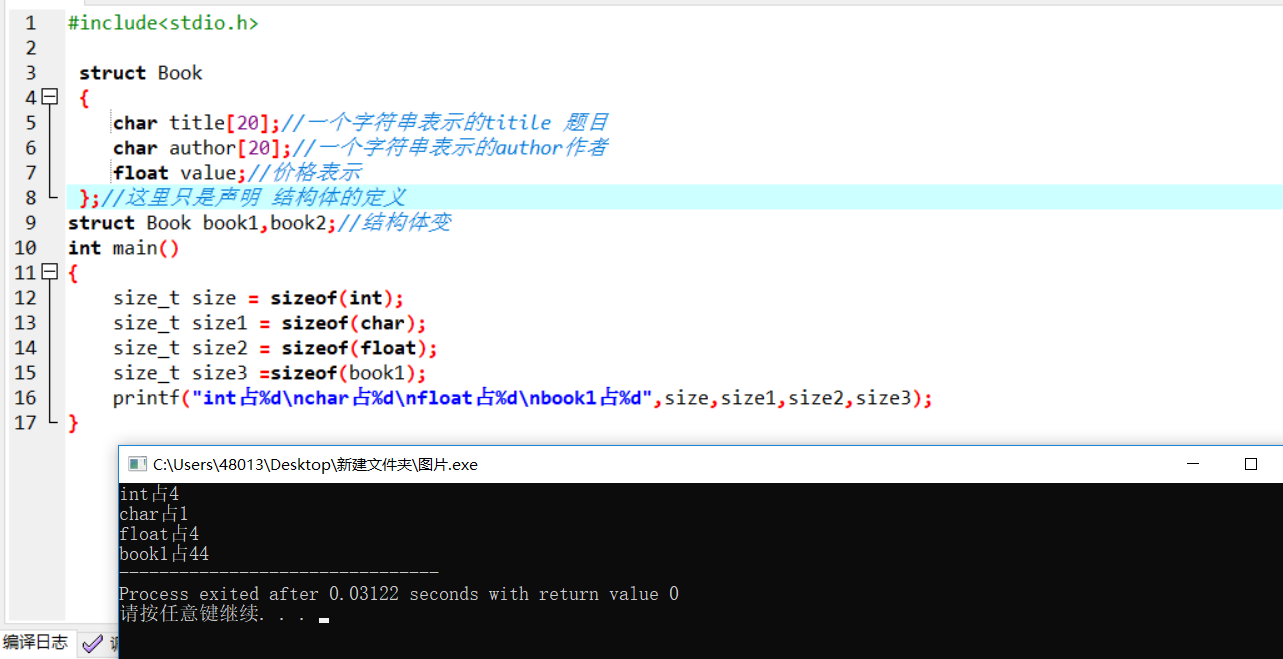1. 结构体整体空间是占用空间最大的成员（的类型）所占字节数的整数倍。
2. 结构体的每个成员相对结构体首地址的偏移量(offset)都是最大基本类型成员字节大小的整数倍，如果不是编译器会自动补齐.

 ``````1 2 3 4 5 6 7 8 `````` ``````struct S1 { char a; int b; double c; };``````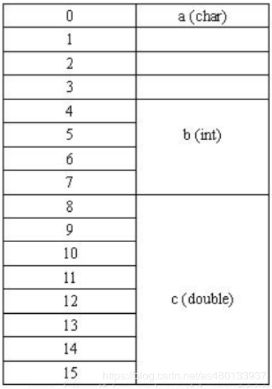## 结构体变量的初始化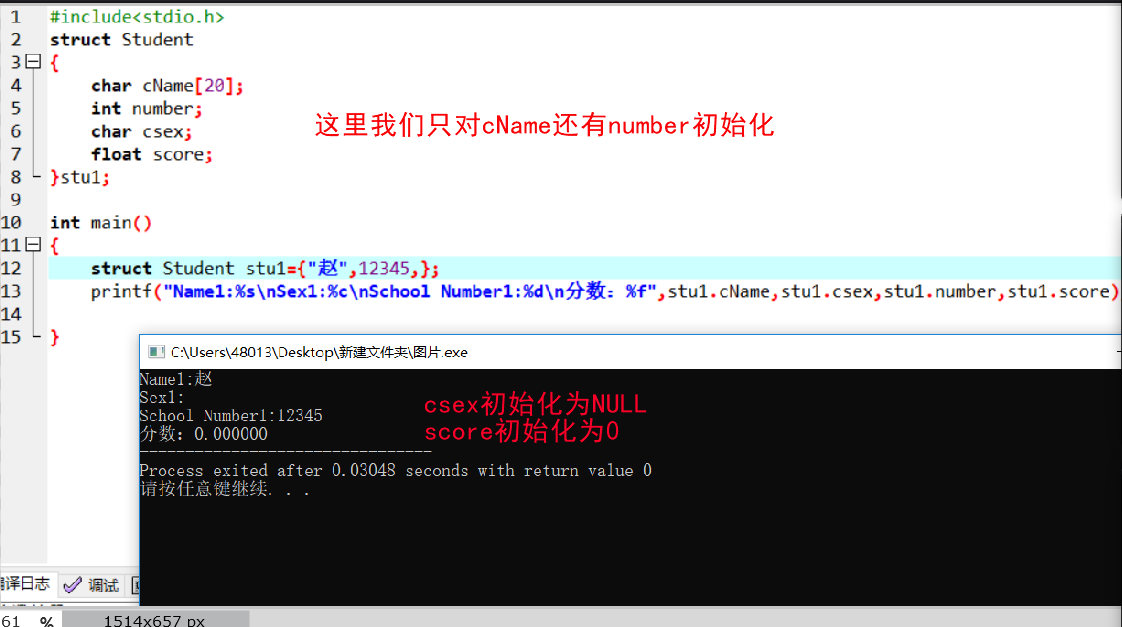`````` 1 2 3 4 5 6 7 8 9 10 11 12 13 14 `````` ``````struct Student { char name; char sex; int number; }stu1={"zhaozixuan",'M',12345}; //或者 struct Student { char name; char sex; int number; }； struct Student stu1={"zhaozixuan",'M',12345};``````

 ``````1 2 3 4 5 `````` ``````stu1.name="王伟"； stu1.sex='M'; stu1.number=12305; //也可用strcpy函数进行赋值 strcpy(stu1.name,"王伟");``````

 ``````1 2 3 4 5 `````` `````` struct Student stu1={ .name="Wang", .number=12345, .sex='W', };//可以对任意变量赋值``````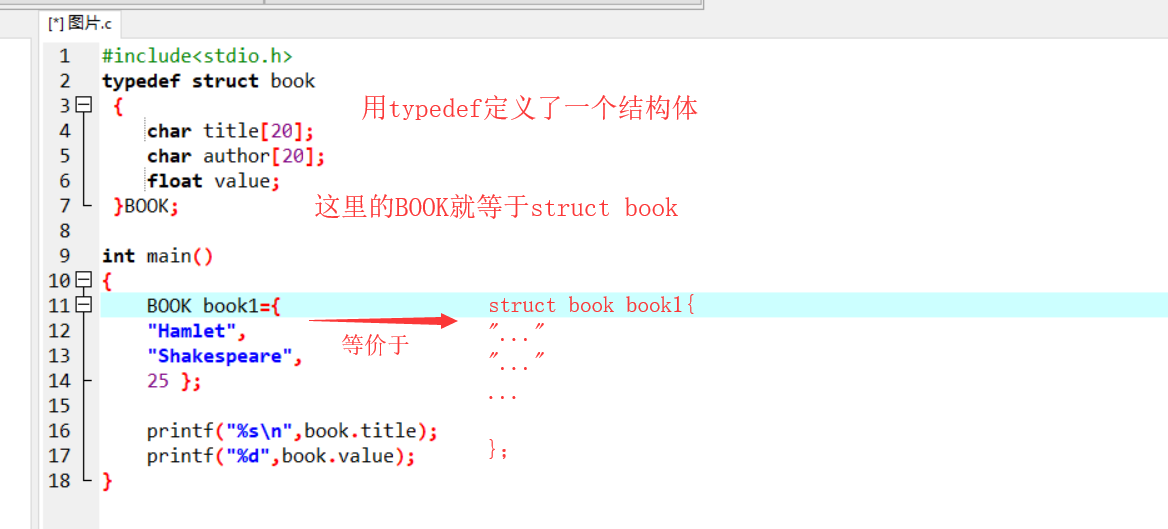## 结构体变量的引用(输出和输入)

• `.`是运算符，在所有运算符优先级中最高
• 如果结构体的成员本身是一个结构体，则需要继续用.运算符，直到最低一级的成员。

 `````` 1 2 3 4 5 6 7 8 9 10 11 12 13 14 `````` ``````struct Student { char name; char sex; int number; struct Date { int year; int month; int day; }birthday; }stu1; printf("%d",stu1.birthday);//这样子是错误的，因为birthday也是一个结构体变量 scanf("%d",&stu1.birthday.month);//正确``````
• 可以引用接头体变量成员的地址,也可以引用结构体变量的地址和

## 结构体数组及其初始化

 `````` 1 2 3 4 5 6 7 8 9 10 11 12 13 14 15 `````` ``````struct Student { char name; char sex; int number; } stu1={ {"zhaozixuan",'M',12345}, {"houxiaohong",'M',12306}, {"qxiaoxin",'W',12546}, {"wangwei",'M',14679}, {"yulongjiao",'W',17857} }; stu1.name//表示stu1的第三个结构变量中姓名的第五个字符 //若初始化时已经是结构体数组全部元素[]中的数可以不写如stu1[]=``````

 ``````1 2 3 4 5 6 7 `````` ``````struct Student stu1； stu1={ {"zhaozixuan",'M',12345}, {"houxiaohong",'M',12306}, {"qxiaoxin",'W',12546} }; //错误``````

1. 使用strcpy或者memset函数进行复制

 ``````1 2 3 `````` ``````char str; strcpy(str,"I love you"); 再用到memset函数时,出现了问题``````

memset `void *memset(void *s,int c,size_t n)` 作用：将已开辟内存空间s的首n个字节的值设为值c。

 ``````1 2 `````` ``````char str memset(str,'a',20);``````

``````int str;
memset(str,1,sizeof(str));``````
1. 用指针

 ``````1 2 `````` ``````char *str; str = "I love you";``````

## 结构体与指针

### 指向结构体变量的指针

 `````` 1 2 3 4 5 6 7 8 9 10 11 12 `````` ``````struct Student { char cName; int number; char csex; }student1; struct Student*p; p=&student1; //若为结构体数组则 struct Student stu1; struct Student*p; p=stu1;//因为stu1为结构体数组而p=stu1直接是指向stu1的首地址，就不用再加&符``````

 ``````1 2 3 4 5 6 `````` ``````p->cName //1 (*p).cName //2 student1.cName//3 p->cName //可以进行正常的运算``````

### 指向结构体数组的指针

 ``````1 2 3 4 `````` ``````struct Student stu1; struct Student*p; p=stu[n]; (++p).number//是指向了结构体数组下一个元素的地址``````

### 结构体成员是指针类型变量

 ``````1 2 3 4 5 6 `````` ``````struct Student { char* Name;//这样防止名字长短不一造成空间的浪费 int number; char csex; }student1;``````

 ``````1 2 3 4 5 6 7 8 `````` ``````struct Student { char* Name; int number; char csex; }stu，*stu； stu.name=(char*)malloc(sizeof(char));//内存初始化``````

 ``````1 2 3 4 5 6 7 8 `````` ``````struct Student { char* Name; int number; char csex; }stu,*stu; stu = (struct student*)malloc(sizeof(struct student));./*结构体指针初始化*/ stu->name = (char*)malloc(sizeof(char));/*结构体指针的成员指针同样需要初始化*/ ``````

### 结构体作为函数参数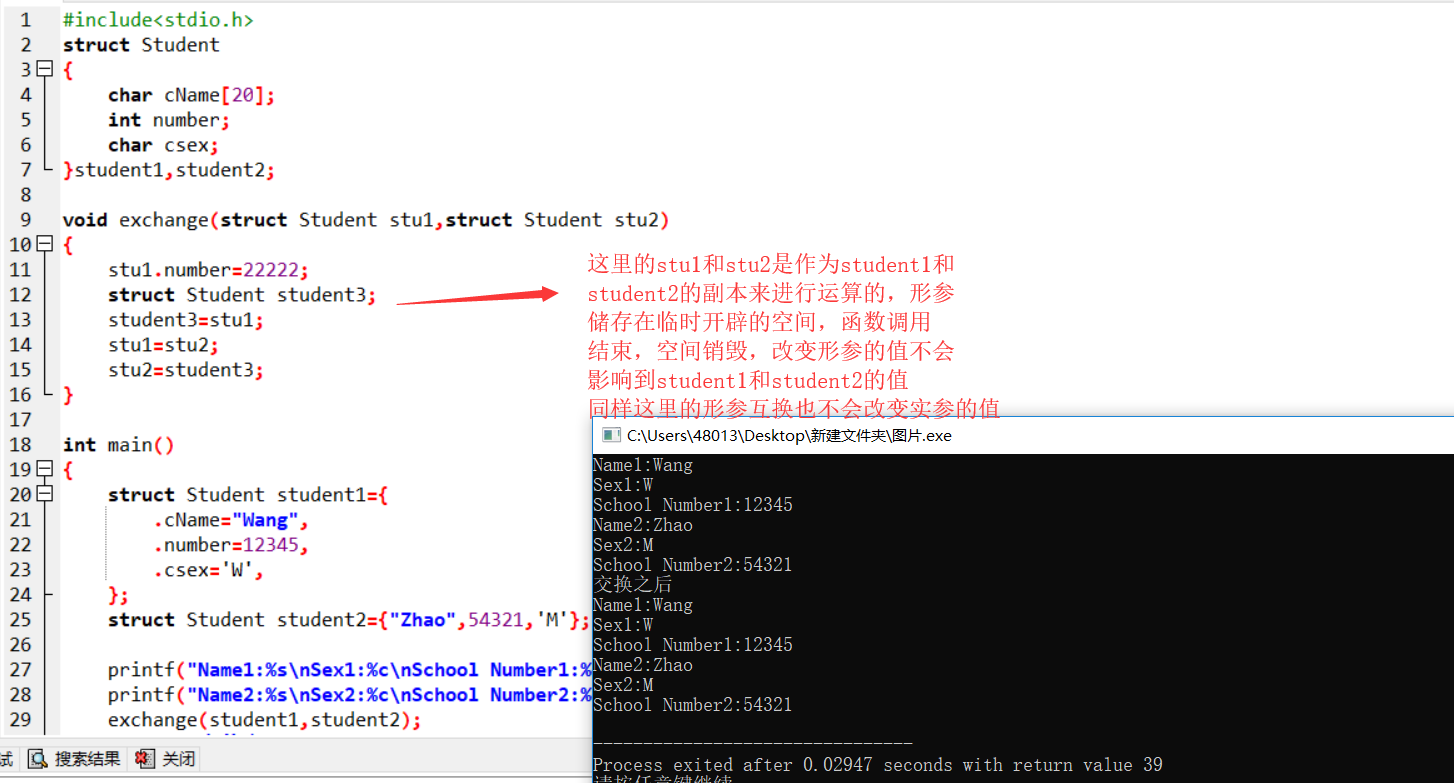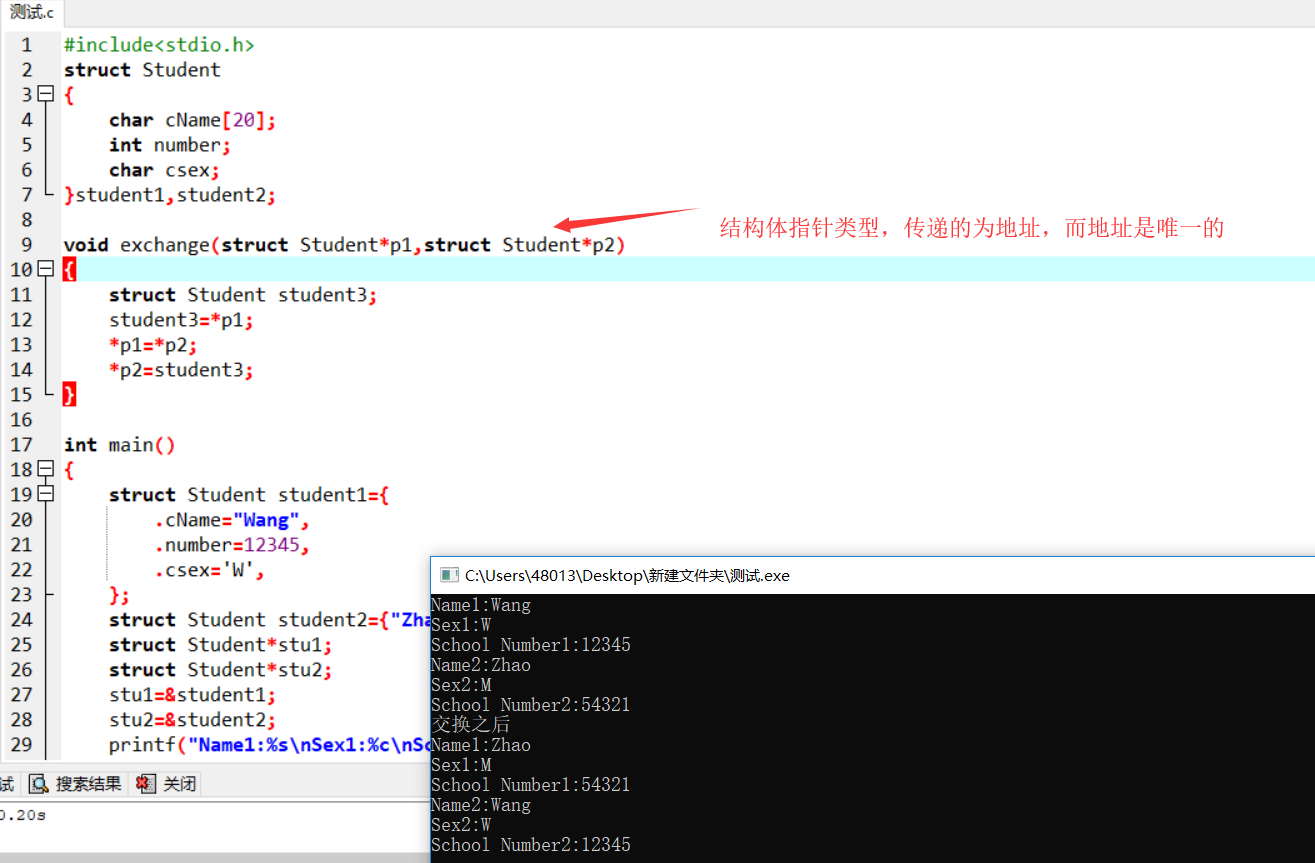`````` 1 2 3 4 5 6 7 8 9 10 11 12 13 14 15 16 17 18 19 20 21 22 23 24 25 26 27 `````` ``````struct Student { char cName; int number; char csex; }student1,student2; struct Student student1={"Wang",12345,'W'}; struct Student student2={"Zhao",54321,'M'}; struct Student*stu1=&student1; struct Student*stu2=&student2; struct Student *student3; student3=stu1; stu1=stu2; stu2=student3;//互换地址 2对于同类型结构体直接互换值就行 struct stu student3; student3=student1; student1=student2; student2=student3; //这里也可以写成应strcmp函数互换 3用memcpy()函数进行互换 4比较笨的方法： 用for循环互换``````

 `````` 1 2 3 4 5 6 7 8 9 10 11 12 13 14 15 16 17 `````` ``````struct Student { char cName; int number; char csex; }stu1; 一般情况下，清空str的方法： 　　str.cName='\0'; 　　str.csex='0'; 　　str.number=0; 　　但是我们用memset就非常方便： 　　memset(&str,0,sizeof(struct Student)); 　　如果是数组： 　　struct Student stu; 　　就是 　　memset(stu,0,sizeof(struct Student)*10);``````